•5星
108.34MB guoruibin123 2021-04-27 06:03:44
•4星
1.35MB m0_52957036 2020-05-30 17:02:35
• 栈应用之行编辑程序(C语言) c语言

void LineEdit(){ Stack S; InitStack(S); char ch; printf("请输入...\n"); fflush(stdout); ch = getchar();//获取输入字符 while (ch != EOF){ ... if (StackEmpty(S)){//空时不能退格
void LineEdit(){
Stack S;
InitStack(S);
char ch;
printf("请输入...\n");
fflush(stdout);
ch = getchar();//获取输入字符
while (ch != EOF){
while (ch != EOF && ch != '\n'){
ELEMTYPE e;
switch (ch){
case '#':
if (StackEmpty(S)){//栈空时不能退格
printf("未输入任何字符.\n");
return;
}
Pop(S, e);//退格（即出栈）
break;
case '@':
if (StackEmpty(S)){//栈空时不能退行
printf("未输入任何字符.\n");
return;
}
ClearStack(S);//退行（即清空栈）
break;
default:
if (StackFull(S)){
printf("最多输入%d个字符\n", MAXSIZE);
return;
}
Push(S, ch);//默认是进栈
break;
}
ch = getchar();//进行下一次输入
}
printStack(S);//先打印栈
fflush(stdout);
ClearStack(S);//再清空栈
if (ch != EOF)//当输入为换行符
ch = getchar();//开始下一行输入
}
ClearStack(S);//输入为EOF(Ctrl+Z)，清空栈，退出程序
}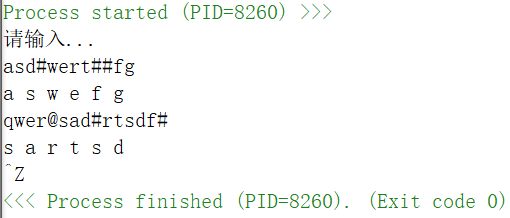展开全文Hackeryuan 2021-03-31 20:20:43
• 简单又实用的栈实现和栈应用（内附实现代码） 数据结构 c++

的概念 在正式讲的概念前，先举个例子，其实就像弹夹，我们把子弹一个一个压进去，开枪的时候最后一个压进去的子弹第一个被打出来。 也有这样的特性：“FILO”（First In，Last Out），先进后出，后进先出...

栈的概念

在正式讲栈的概念前，先举个例子，栈其实就像弹夹，我们把子弹一个一个压进去，开枪的时候最后一个压进去的子弹第一个被打出来。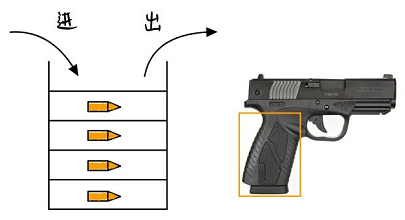栈也有这样的特性：“FILO”（First In，Last Out），先进后出，后进先出。我们不难发现栈 ⊂ \subset 数组或链表，那么为什么我们还需要栈而不直接使用数组或链表呢？因为在某些情况下我们不想让别人用其他的除了我们限定的方法处理数据。当我们要处理的数据满足上面FILO特性而且只被允许在一端push或pop时，栈是个很好的选择。

栈的实现

栈的链表实现跟数组实现差不多，区别在于数组实现需要提前初始化数组的大小，而链表实现可以实现动态扩容。所以我只写了个数组实现，当然数组也可以动态扩容，当栈满了的时候，先建一个比如两倍大的数组，再把原本的所有数据一次性拷贝，最后把原本的内存释放。原本没有写动态扩容代码的想法，写到这觉得干脆再补充一下吧。代码实现放在最后了。

栈的应用

平衡符号

一般来说，括号什么都是要一一对应，有开有闭（左括号’(‘和右括号 ‘)‘要一一对应，不能是’(‘对应’]’，只有’(‘没有’)‘也是不对的），栈就可以用来判断符号是否平衡。实现很简单，检测到’(’, ‘)’, ‘{’, ‘}’, ‘[’, ‘]’ 就把它们入栈，检测到对应的就将它们出栈，不对应的就报错，如果最后栈不为空也报错。

调用函数

函数的调用类似于平衡符号，可以这样理解，调用函数类似于左括号，函数运行完的返回类似于右括号。每调用一个函数，就会将所需要储存的信息（专有名词为活动记录或栈帧）入栈，返回后，就将这些信息出栈。

栈的数组版代码实现

MyStack.h

//
// Created by Sky on 2021/3/14.
//

#ifndef DATA_STRUCTURE_PRACTICES_MYSTACK_H
#define DATA_STRUCTURE_PRACTICES_MYSTACK_H
#include <iostream>
using namespace std;

class MyStack{
public:
MyStack(int n);
bool isEmpty();
bool isFull();
bool push(int num);
bool pop();
int top();
~MyStack();

private:
void expand();
int* data;
int capacity;
int size;
};

#endif //DATA_STRUCTURE_PRACTICES_MYSTACK_H

MyStack.cpp

//
// Created by Sky on 2021/3/14.
//

#include "MyStack.h"
MyStack::MyStack(int n) {
int* temp = new int[n];
data = temp;
capacity = n;
size = 0;
cout << "Stack has been created" << endl;
}
MyStack::~MyStack() {
delete data;
cout << "Stack has been deleted" << endl;
}

bool MyStack::isFull() {
if(capacity == size){
expand();
return true;
}
return false;
}

bool MyStack::isEmpty() {
if(size == 0)
return true;
return false;
}

bool MyStack::push(int num) {
if (isFull())
return false;
data[size++] = num;
return true;
}

bool MyStack::pop() {
if(isEmpty())
return false;
--size;
return true;
}

int MyStack::top() {
if (isEmpty())
return -1;
//    cout << data[size] << endl;
return data[size-1];
}

void MyStack::expand() {
int* temp = new int [2*capacity];
for (int i = 0; i < capacity; i++){
temp[i] = data[i];
}
delete data;
data = temp;
capacity *= 2;
}

main.cpp

#include <iostream>
#include "MyStack.h"

using namespace std;

int main() {
MyStack s(5);
cout << boolalpha << "Can we pop? " << s.pop() << endl;    // 测试空的情况下能不能pop
cout << boolalpha << "Is stack empty? " << s.isEmpty() << endl;// 测试空的情况下的返回值
cout << s.top() << endl;    // 测试空的情况下能不能返回栈顶
s.push(111);             // 测试push
cout << boolalpha << "Is stack empty? " << s.isEmpty() << endl;// 测试非空的情况下的返回值
cout << s.top() << endl;    // 测试非空的情况下能不能返回栈顶
cout << boolalpha << "Can we pop? " << s.pop() << endl;    // 测试非空的情况下能不能pop
s.push(2);
s.push(3);
s.push(4);
s.push(5);
s.push(6);
cout << boolalpha << "Is stack full? " << s.isFull() << endl; // 测试是否满了，满了之后会返回真，同时自动扩容
cout << boolalpha << "Is stack full? " << s.isFull() << endl; // 测试扩容后是否还会满
cout << boolalpha << "Can we push? " << s.push(7)  << endl; //测试满了还能不能push

return 0;
}

展开全文skywuuu 2021-03-14 09:31:18
• 栈应用：中缀表达式实现 像我们平时数学计算中的计算公式使用的就是中缀表达式的形式，即符号位位于两个数值中间，在计算机中为了实现对这种中缀表达式的运算，一般使用栈这种数据结构。 思路分析 1.我们需要两个栈 ...

栈应用：中缀表达式实现

像我们平时数学计算中的计算公式使用的就是中缀表达式的形式，即符号位位于两个数值中间，在计算机中为了实现对这种中缀表达式的运算，一般使用栈这种数据结构。
思路分析

1.我们需要两个栈 ，一个栈存放数值位，一个栈存放符号，当我们扫描表达式字符串时，我们将扫描的字符分别放入两个栈中。
2.在我们扫描的过程中，我们需要将运算符优先级较大的先计算出来，类如 * ，/等，当我们扫描结束后，栈中的运算符只剩下优先级小或者是即使是较大的也不影响后续的计算的。
3.最后我们只需要将这些优先级较小的计算即可，
注意
在最后计算过程中，要对减号在左边的要格外考虑，由于栈是一种先进后出的数据结构，当减号在左边时，容易出现 被减数竟然与后一位相加扩大被减数的情况，类如9-4+2，当我们不注意时 ，就会出现9-6的情况，先计算的后两位相加，在计算的相减。
代码实现

import calculator.CalculatorStack;

/** @Author: 云萧YYY @DateTime: 2021/08/10 @Description: 使用stack 完成对四则公式的计算 */
public class Calculator {

public static void main(String[] args) {
//
String expresion = "90+5*2-20*2";
int num1 = 0;
int num2 = 0;
int res = 0;
int index = 0;
char ch = 0;
String keepNum = ""; //临时保存的数字
CalculatorStack numStack = new CalculatorStack(10);
CalculatorStack operStack = new CalculatorStack(10);
// 扫描expresion 压栈
while (true) {
// 获取字符
ch = expresion.substring(index, index + 1).charAt(0);
// 判断是否为运算符
if (operStack.isOperator(ch)) {
if (!operStack.isEmpty()) {
// 比较操作符的优先级
if (operStack.priority(ch) <= operStack.priority(operStack.peek())) {
// 弹出两个数字做运算
num1 = numStack.pop();
num2 = numStack.pop();
res = numStack.cal(num1, num2, operStack.pop());
// 结果压栈
numStack.push(res);
// 运算符压栈
operStack.push(ch);
} else {
operStack.push(ch);
}
} else {
// 若oper为空直接压栈
operStack.push(ch);
}
} else {
// 是数字，直接压栈，考虑数字是多位数字
keepNum += ch;
// 最后一位
if (index == expresion.length() - 1) {
numStack.push(Integer.parseInt(keepNum));
} else {
// 提前看一位
if (operStack.isOperator(expresion.substring(index + 1, index + 2).charAt(0))) {
numStack.push(Integer.parseInt(keepNum));
keepNum = "";
}
}
//  numStack.push(ch - 48);
}
index++;
if (index >= expresion.length()) {
break;
}
}
// 计算结果
while (!operStack.isEmpty()) {

int temp1 = numStack.pop();
int temp2 = numStack.pop();
int opertemp = operStack.pop();
if (operStack.size() == 0) {
res = numStack.cal(temp1, temp2, opertemp);
numStack.push(res);
break;
}
if (operStack.priority(opertemp) < operStack.priority(operStack.peek())) {
int opertemp2 = operStack.pop();
int temp3 = numStack.pop();
res = numStack.cal(temp2, temp3, opertemp2);
numStack.push(res);
numStack.push(temp1);
operStack.push(opertemp);
} else {
res = numStack.cal(temp1, temp2, opertemp);
// 结果压栈
numStack.push(res);
}
}
System.out.printf("表达式%s=%d", expresion, numStack.pop());
}
}
`

展开全文qq_44789195 2021-08-10 13:31:05
• 数据结构-栈及栈的应用 数据结构 算法

的概述 式一种重要的线性结构，属于一种操作受限的线性表 (stack) 是限定尽在表尾进行插入或删除操作的线性表 表尾端称为栈顶(top)，表头端称为底(bottom) 的元素先进后出(后进先出)，即最先进入栈的...

目录

栈的概述

部分算法分析

顺序栈的表示和实现

global.h

Stack.h

StackTest.cpp

运行结果

栈的应用

数的任意进制转换

括号匹配检验

栈的概述

•  栈是一种重要的线性结构，属于一种操作受限的线性表
• 栈(stack) 是限定仅在表尾进行插入或删除操作的线性表
• 表尾端称为栈顶(top)，表头端称为栈底(bottom)
• 栈的元素遵循先进后出(后进先出)，即最先进入栈的元素最后出栈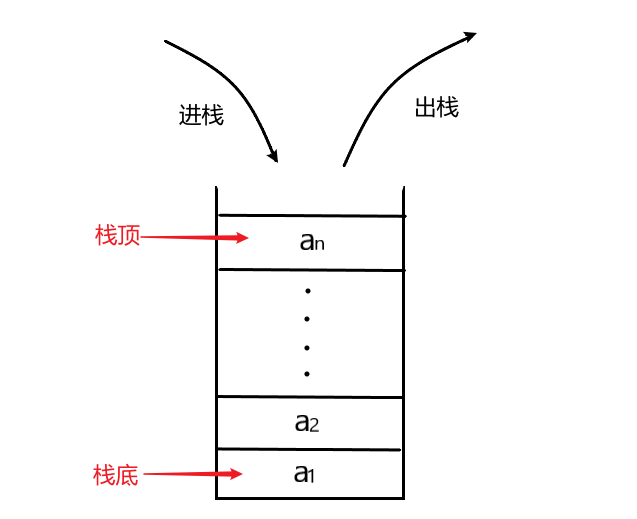部分算法分析

• 栈的初始化
//栈的初始化(构造一个空的栈)
Status InitStack(Stack& S) {
//为栈开辟空间
S.base = (SElemType*)malloc(STACK_INIT_SIZE * sizeof(SElemType));
if (!S.base)
return ERROR;
//当top指针与base指针指向地址时，栈为空栈
S.top = S.base;
S.stacksize = STACK_INIT_SIZE;
return OK;
}

顺序栈，即栈的顺序存储结构，利用一组地址连续的存储单元依次存放自栈底到栈顶的数据元素，注意：top指针所指的地址并不是存储栈顶数据元素的，而是栈顶数据元素的上一个空间地址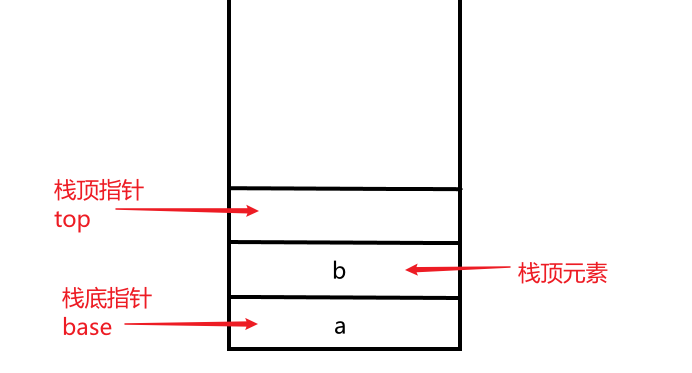•  数据入栈操作
//数据元素data入栈操作
Status PushStack(Stack& S, SElemType data) {
//判断栈内的数据是否已满，已满追加新的存储空间
if (S.top - S.base >= S.stacksize) {
//为栈追加新的空间
SElemType* newbase;
newbase = (SElemType*)realloc(S.base,(S.stacksize + STACK_INCREMENT)*sizeof(SElemType));
if (newbase)
return ERROR;
S.base = newbase;
//防止出现新开辟的空间中存储了其它程序的数据被覆盖
S.top = S.base + S.stacksize;
S.stacksize += STACK_INCREMENT;
}
if(S.top != NULL)
*(S.top++) = data;
return OK;
}

追加存储空间使用的是realloc(void *ptr, size_t size) 函数

ptr指针指向一个要重新分配内存的内存块，如果为空指针，则会分配一个新的内存块，且函数返回一个指向它的指针

size内存块的新的大小，以字节为单位。如果大小为 0，且 ptr 指向一个已存在的内存块，则 ptr 所指向的内存块会被释放，并返回一个空指针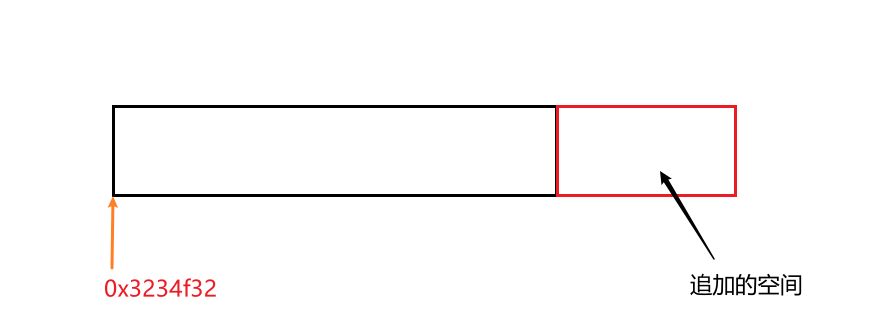情况1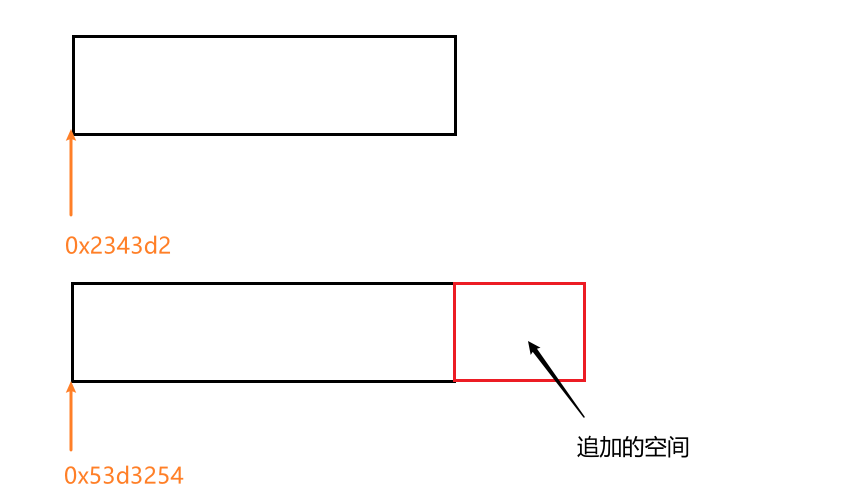情况2

在空间扩大的过程中，如果在原地址后有足够的空间追加，则直接在原空间后扩大空间，如果原地址后没有足够的空间追加，则会在新的一块地址区域直接开辟一块size大小的地址空间

顺序栈的表示和实现

• global.h
• Stack.h
• StackTest.cpp

global.h

相关头文件的引用，以及相应全局变量、常量的声明

#pragma once

#include<iostream>
#include<string.h>
#include<stdlib.h>
#include<stdio.h>
#include<windows.h>

using namespace std;

#define TRUE 1

#define FALSE 0

#define OK 1

#define ERROR 0

#define INFEASIBLE -1

#define EQ(a,b) ((a) == (b))

#define LT(a,b) ((a) < (b))

#define LQ(a,b) ((a) <= (b))

#define MT(a,b) ((a) > (b))

#define MQ(a,b) ((a) >= (b))

typedef int Status;

Stack.h

顺序栈的定义，以及顺序栈的相关操作算法

#pragma once
#include"global.h"

#define STACK_INIT_SIZE 100
#define STACK_INCREMENT 10
#define SElemType int

//栈的定义
typedef struct {
SElemType* base;  //栈底指针
SElemType* top;   //栈顶指针
int stacksize;    //栈的空间大小
}Stack;

//栈的初始化(构造一个空的栈)
Status InitStack(Stack& S) {
//为栈开辟空间
S.base = (SElemType*)malloc(STACK_INIT_SIZE * sizeof(SElemType));
if (!S.base)
return ERROR;
//当top指针与base指针指向地址时，栈为空栈
S.top = S.base;
S.stacksize = STACK_INIT_SIZE;
return OK;
}

//空栈的数据赋值操作
Status InitStackData(Stack& S) {
//判断该栈是否为空栈
if (S.top != S.base) {
cout << "该栈不是空栈" << endl;
return ERROR;
}
cout << "输入插入存储数据的个数:" << endl;
int nums = 0;
cin >> nums;
cout << "输入" << nums << "个数据:" << endl;
for (int i = 0; i < nums; i++) {
cin >> *(S.top++);
}
return OK;
}

//栈的数据遍历
void ShowStack(Stack S) {
cout << "栈内的数据元素有:" << endl;
while (S.top != S.base) {
cout << *(--S.top) << " ";
}
cout << endl;
}

//判断栈是否为空栈
Status StackEmpty(Stack S) {
if (S.top == S.base)
return OK;
return ERROR;
}

//栈的销毁
Status DestroyStack(Stack& S) {
S.top = S.base;
free(S.base);
cout << "栈销毁成功" << endl;
return OK;
}

//将栈置为空栈
Status ClearStack(Stack& S) {
S.top = S.base;
cout << "栈置为空栈成功" << endl;
return OK;
}

//输出栈内的数据个数
int StackLength(Stack S) {
int length = S.top - S.base;
return length;
}

//获取栈顶的数据
void GetTop(Stack S) {
cout << "栈顶的数据为:" << *(S.top - 1) << endl;
}

//数据元素data入栈操作
Status PushStack(Stack& S, SElemType data) {
//判断栈内的数据是否已满，已满追加新的存储空间
if (S.top - S.base >= S.stacksize) {
//为栈追加新的空间
SElemType* newbase;
newbase = (SElemType*)realloc(S.base,(S.stacksize + STACK_INCREMENT)*sizeof(SElemType));
if (newbase)
return ERROR;
S.base = newbase;
//防止出现新开辟的空间中存储了其它程序的数据被覆盖
S.top = S.base + S.stacksize;
S.stacksize += STACK_INCREMENT;
}
if(S.top != NULL)
*(S.top++) = data;
return OK;
}

//将栈顶数据元素出栈，并记录出栈的数据元素
Status PopStack(Stack& S, SElemType& data) {
//判断栈是否为空，不为空则删除栈顶数据元素(出栈)
if (S.top == S.base)
return ERROR;
data = *(--S.top);
return OK;
}

StackTest.cpp

#include"Stack.h"

int main() {
Stack stack;
InitStack(stack);
InitStackData(stack);
ShowStack(stack);
int length = StackLength(stack);
cout << "栈内的元素个数为:" << length << endl;
SElemType data;
GetTop(stack);
cout << "入栈操作,请输入入栈的数据:" << endl;
cin >> data;
PushStack(stack,data);
ShowStack(stack);
cout << "------出栈操作------" << endl;
PopStack(stack, data);
cout << "出栈的数据是:" << data << endl;
ShowStack(stack);
}

运行结果

输入插入存储数据的个数:
5
输入5个数据:
12 23 43 21 56
栈内的数据元素有:
56 21 43 23 12
栈内的元素个数为:5
栈顶的数据为:56
入栈操作,请输入入栈的数据:
87
栈内的数据元素有:
87 56 21 43 23 12
------出栈操作------
出栈的数据是:87
栈内的数据元素有:
56 21 43 23 12

F:\DataStructureForC++\Project\Debug\Project1.exe (process 5248) exited with code 0.

• 数的任意进制转换
• 括号匹配检验
• 表达式求值

数的任意进制转换

将一个十进制数N与其他d进制数转换，基于下列原理

N = (N div d) × d + N mod d (div为整除运算，mod为求余运算)

/*
* 数的任意进制转换
* 用户输入任意非负正整数，再输入一个进制数
*/
void NumberConversion(int number,int baseNumber) {
//判断输入的数是否为正整数
if (number < 0) {
cout << "输入的数不能为负数" << endl;
exit(0);
}
Stack stack;
SElemType data;
InitStack(stack);
cout << number << "转化" << baseNumber << "进制后的数为:" << endl;
while (number) {
PushStack(stack, number % baseNumber);
number = number / baseNumber;
}
while (!StackEmpty(stack)) {
PopStack(stack, data);
cout << data;
}
cout << endl;
}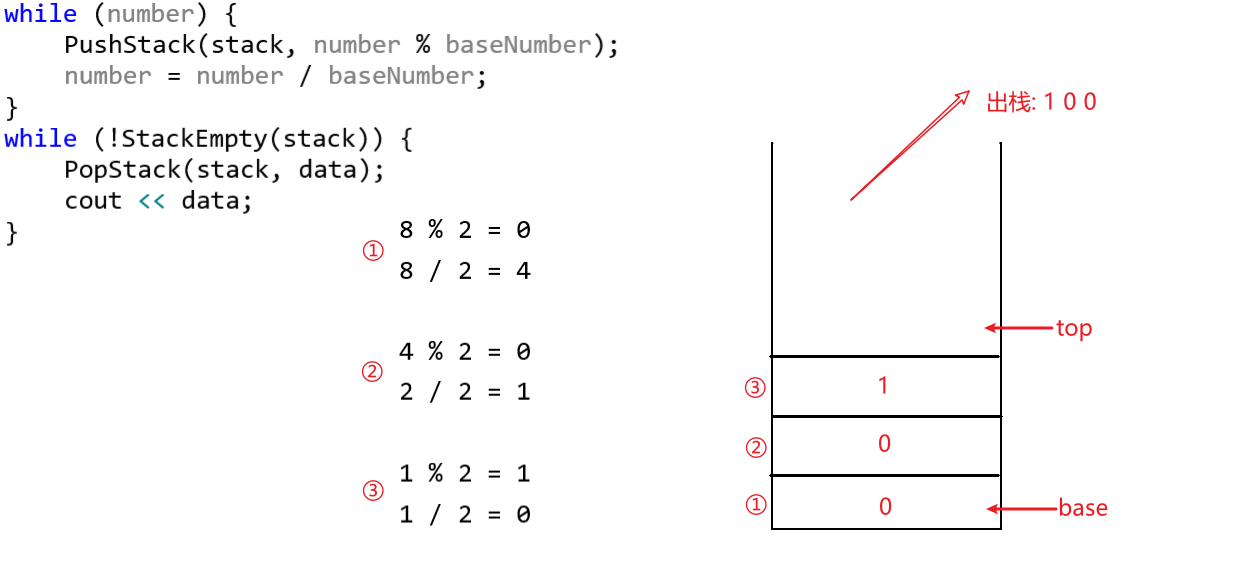运行结果

请输入一个非负的十进制整数
8
请输入一个进制数:
2
8转化2进制后的数为:
1000

请输入一个非负的十进制整数
89
请输入一个进制数:
8
89转化8进制后的数为:
131

括号匹配检验

假设表达式中允许包含两种括号：圆括号（）和方括号 [ ] ，其嵌套顺序随意，检验括号嵌套的格式是否正确

/*
* 括号匹配检验
* 用户输入方括号和圆括号，检验括号是否匹配
* charArray数组以'#'作为最后一个数据元素(循环终止条件)
*/
void BracketMatch(char charArray[]) {
Stack stack;
InitStack(stack);
SElemType c;
while (*charArray != '#') {
switch ((*charArray)) {
case ')':
PopStack(stack, c);
if (c != '(') {
cout << ") 括号不匹配" << endl;
exit(0);
}
break;
case ']':
PopStack(stack, c);
if (c != '[') {
cout << "] 括号不匹配" << endl;
exit(0);
}
break;
default:
//如果不是右括号，将该括号进栈
PushStack(stack, *(charArray));
}
charArray++;
}
cout << "括号匹配成功" << endl;
}

运行结果

输入的括号为:
( [ ] ) 括号匹配成功

输入的括号为:
( [ ( ] ] ) ] 括号不匹配

F:\DataStructureForC++\Project\Debug\Project1.exe (process 2124) exited with code 0.

注意:将Stack.h头文件中的#define SElemType int改为char

括号匹配函数中，如果遇到左括号则入栈，遇到右括号则进入switch( )语句，将栈内的栈顶元素出栈(该元素为最内层的括号)，检查括号是否匹配

展开全文weixin_47467620 2021-07-18 11:10:23
• weixin_51601437 2021-07-29 19:33:50
• 栈的应用（c语言） 数据结构 c语言

weixin_45774615 2021-01-19 07:50:58
• 栈的几个实例应用 数据结构

heitong_fu 2021-08-24 19:12:07
• 栈应用—字符串括号匹配问题 数据结构 算法

ltx1472580369 2021-04-13 18:12:21
• 栈的应用-括号匹配问题 数据结构

weixin_45808737 2021-03-15 20:00:28
• Python实现栈及其简单应用 数据结构

weixin_45859741 2021-08-03 10:00:01
• weixin_30709179 2021-03-16 02:49:26
• 栈的应用练习题 数据结构

yuwoxinanA3 2021-11-04 15:39:23
• 栈的应用-算数表达式求值 数据结构 c++ 算法

QWDWQWQ 2021-08-04 10:11:06
• 顺序栈和链栈以及他们的应用 数据结构

lizefeng1998 2021-04-15 16:21:58
• 【数据结构】【C语言】 栈的应用之进制转换 数据结构 c语言 算法

Mr_VK 2021-09-08 19:14:29
• qq_36314864 2021-03-30 22:43:46
• the_Milky 2021-01-24 18:22:26
• Xcodd 2021-04-30 10:36:35
• 栈应用之平衡符号 - 亚特兰缇斯的个人空间 - OSCHINA - 中文开源技术交流社区 java平衡符号

weixin_42138545 2021-03-11 12:40:15
• java中栈的应用 java 编程语言

qq_43413774 2021-03-28 11:08:11
• 栈的应用——表达式求值 数据结构

weixin_43715360 2021-07-30 15:29:39
• 数据结构--栈的基本概念与应用 数据结构 顺序栈 链栈

qq_34666857 2021-11-06 10:12:27
• 栈的应用之递归 c语言 递归算法 数据结构

weixin_45463343 2021-06-01 14:51:20
• m0_46663240 2021-02-01 16:31:17
• 栈的使用场景和介绍 数据结构

qq_45020745 2021-04-08 11:02:51
• 栈及其基本应用 算法 数据结构

qq_52928436 2021-08-01 19:03:58
• 栈的应用——括号匹配 编译器 python 算法 数据结构

weixin_45748734 2021-09-28 11:01:50
• 数据结构 实验二 栈的应用 数据结构 链表 算法

weixin_51733609 2021-11-04 19:49:20
• 第三章栈和队列——3.2：栈的应用举例 算法 数据结构 c语言

Hardenman 2021-06-06 22:30:26
• 数据结构与算法之-----栈的应用（二） 数据结构 算法

sixibiheye 2021-08-20 10:43:46...